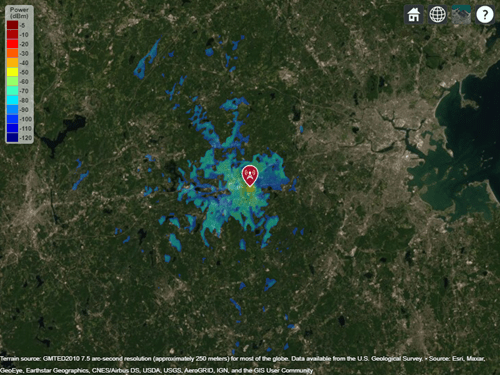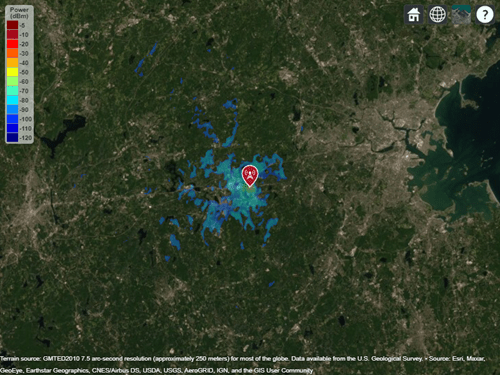# LongleyRice

Longley-Rice propagation model

Since R2019b

## Description

Model the behavior of electromagnetic radiation from a point of transmission over irregular terrain, including buildings, by using the Longley-Rice model, also known at the Irregular Terrain Model (ITM) . Represent the model by using a `LongleyRice` object.

The Longley-Rice model:

• Is designed for frequencies from 20 MHz to 20 GHz, distances from 1 to 2000 km, and antenna heights from 0.5 to 3000 m.

• Calculates path loss from free-space loss, terrain and obstacle diffraction, ground reflection, atmospheric refraction, and tropospheric scatter.

• Provides path loss estimates by combining physics with empirical data.

## Creation

Create a `LongleyRice` object by using the `propagationModel` function.

## Properties

expand all

Polarization of transmitter and receiver antennas, specified as `"horizontal"` or `"vertical"`. This object assumes both antennas have the same polarization.

Data Types: `char` | `string`

Conductivity of the ground, specified as a scalar in siemens per meter (S/m). The model uses this value to calculate path loss due to ground reflection and diffraction. The default value corresponds to average ground.

Data Types: `double`

Real relative permittivity of the ground, specified as a scalar. Real relative permittivity is expressed as the real part of the ratio of complex absolute material permittivity to the absolute permittivity of vacuum. The model uses this value to calculate the path loss due to ground reflection and diffraction. The default value corresponds to average ground.

Data Types: `double`

Atmospheric refractivity near the ground, specified as a scalar in N-Units. The model uses this value to calculate the path loss due to refraction through the atmosphere and tropospheric scatter. The default value corresponds to average atmospheric conditions.

Data Types: `double`

Radio climate zone, specified as one of these options:

• `"continental-temperate"`

• `"equatorial"`

• `"continental-subtropical"`

• `"maritime-subtropical"`

• `"desert"`

• `"maritime-over-land"`

• `"maritime-over-sea"`

The model uses this value to calculate the variability due to changing atmospheric conditions. The default value corresponds to average atmospheric conditions in a particular climate zone.

Data Types: `char` | `string`

Time variability tolerance level of the path loss, specified as a scalar in the range [0.001, 0.999]. Time variability represents long-term variability (including atmospheric conditions) but neglects short-term variability such as fast multipath fading. This value gives the required system reliability expressed as the fraction of time during which the actual path loss is expected to be less than or equal to the model prediction.

Data Types: `double`

Situation variability tolerance level of the path loss, specified as a scalar in the range [0.001, 0.999]. Situation variability occurs due to uncontrolled or hidden random variables. This value gives the required system confidence expressed as the fraction of similar situations for which the actual path loss is expected to be less than or equal to the model prediction.

Data Types: `double`

## Object Functions

 `pathloss` Path loss of radio wave propagation `add` Add propagation models

## Examples

collapse all

Display the coverage area for a transmitter using the Longley-Rice model.

```pm = propagationModel("longley-rice"); tx = txsite("Name","Apple Hill","Latitude",42.3001,"Longitude",-71.3604); coverage(tx,pm,"SignalStrengths",-100:-5)```Increase the time and situation variability tolerance levels from `0.5` (the default) to `0.9`. Display the coverage area for the transmitter using the updated propagation model.

```pm.TimeVariabilityTolerance = 0.9; pm.SituationVariabilityTolerance = 0.9; coverage(tx,pm,"SignalStrengths",-100:5)```The coverage area is smaller for the model with higher variability tolerance levels.